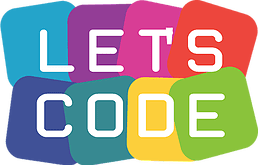Introduction to JavaScript – Libraries – Instance methods, by definition, require that you create an instance before you can use them. What if you want to call a method without an instance? That’s where JavaScript libraries come in. Libraries contain methods that you can call without creating an instance.

One such collection contains mathematical methods, aptly named the Math library.

Let’s see how you call the .random() method from the Math library:

```console.log(Math.random()); // random number between 0 and 1
```

In the example above, we called the .random() method by appending the library name with a period, the name of the method, and opening (() and closing ()) parentheses. This method returns a random number between 0 and 1. This code prints a random number between 0 and 1.

To generate a random number between 0 and 50, we could multiply this result by 50, like so:

```Math.random() * 50;
```

The answer in the example above will most likely be a decimal. To ensure an answer is a whole number, JavaScript provides a built-in method called Math.floor(). Math.floor() takes a decimal number, and rounds down to the nearest whole number. You can use Math.floor() to round a random number like this:

```Math.floor(Math.random() * 50);
```

In this case:

1. Math.random generates a random number between 0 and 1.
2. We then multiply that number by 50, so now we have a number between 0 and 50.
3. Then, Math.floor rounds the number down to the nearest whole number.Play with the code below to continue practising your coding skills with math.random and math.floor. Try to make your program generate a random number from 0 to 99.

# Related Videos:See the JavaScript Glossary on Math Library

Online JavaScript Compiler. Code on the go.

JavaScript Glossary

Systematic approach to Problem Solving

Methods of teaching programming

Alice Teaches OOP (Glossary of useful terms)

Introduction to JavaScript – Control Flow: True and False values

My little pony learning game in VB.NET

Introduction to JavaScript – Review Types and Operators

Introduction to JavaScript – Create a Variable: const

Introduction to JavaScript – Create a Variable: let

Who is this Android App Development course for?

Hello World Android app built with Android Studio

Introduction to JavaScript – Control Flow: if/else Statements

Android Studios

Introduction to JavaScript – Variables: Undefined

Why do most sites use cookies?